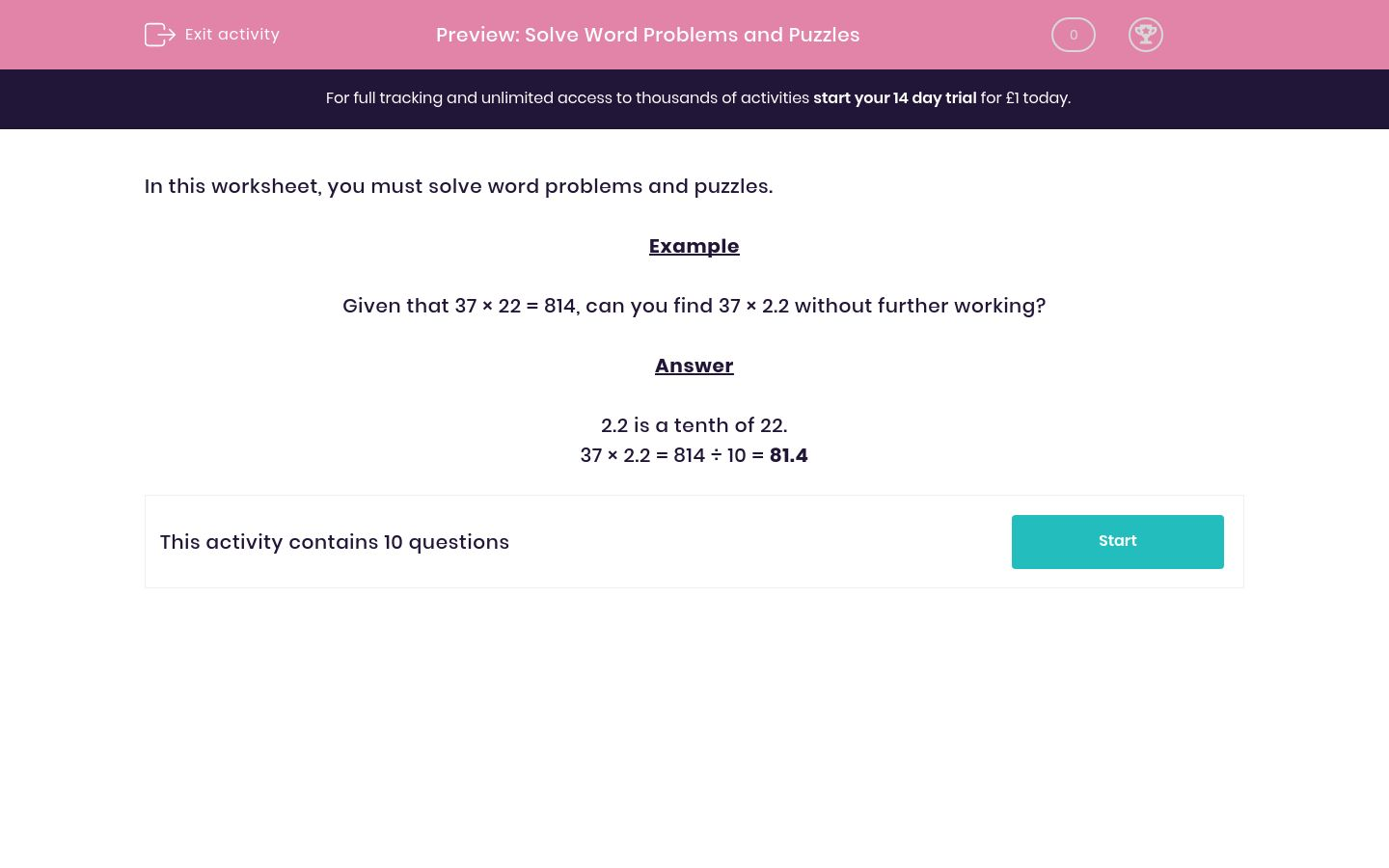# Solve Word Problems and Puzzles

In this worksheet, students resolve word problems and puzzles allowing them to revise and practise a range of mathematical processes for measures, percentages, decimals, fractions and wider number knowlege.Key stage:  KS 2

Curriculum topic:   Maths and Numerical Reasoning

Curriculum subtopic:   Word Problems

Difficulty level:#### Worksheet Overview

In this worksheet, you must solve word problems and puzzles.

Example

Given that 37 × 22 = 814, can you find 37 × 2.2 without further working?

2.2 is a tenth of 22.

37 × 2.2 = 814 ÷ 10 = 81.4

### What is EdPlace?

We're your National Curriculum aligned online education content provider helping each child succeed in English, maths and science from year 1 to GCSE. With an EdPlace account you’ll be able to track and measure progress, helping each child achieve their best. We build confidence and attainment by personalising each child’s learning at a level that suits them.

Get started